### Chapter 1: Solid State Balbharati solutions for Chemistry 12th Standard HSC Maharashtra State Board chapter 1 - Solid State 2020 - 2021

Chapter 1: Solid State

Balbharati solutions for Chemistry 12th Standard HSC Maharashtra State Board chapter 1 - Solid State [Latest edition]

# Choose the most correct answer.

## Molecular solids are _______.

crystalline solids

amorphous solids

ionic solids

metallic solids

Pure Si

Si doped with As

Si doped with Ga

Ge doped with In

## In Frenkel defect, _______.

electrical neutrality of the substance is changed

density of the substance is changed

both cation and anion are missing

overall electrical neutrality is preserved

68 %

74 %

32 %

26 %

2

4

6

8

## Which of the following is CORRECT?

Four spheres are involved in the formation of a tetrahedral void.

The centres of spheres in octahedral voids are at the apices of a regular tetrahedron.

If the number of atoms is N, the number of octahedral voids is 2N.

If the number of atoms is N/2, the number of tetrahedral voids is N.

## A compound forms hcp structure. Number of octahedral and tetrahedral voids in 0.5 mole of substance is respectively _______.

3.011 × 1023, 6.022 × 1023

6.022 × 1023, 3.011 × 1023

4.011 × 1023, 2.011 × 1023

6.011 × 1023, 12.022 × 1023

205 pm

185 pm

260 pm

175 pm

# Answer the following in one or two sentences.

## What are the types of particles in each of the four main classes of crystalline solids?

The type of particles in each of the four main classes of crystalline solids are:

Ionic solids: Constituent particles are cations and anions.

Covalent network solids: Constituent particles are atoms.

Molecular solids: Constituent particles are monoatomic or polyatomic molecules.

Metallic solids: Constituent particles are metallic ions in a sea of electrons.

# Answer the following in one or two sentences.

## Which of the three types of packing used by metals makes the most efficient use of space and which makes the least efficient use?

Out of the three types of packing, face-centered cubic (or ccp or hcp) lattice makes the most efficient use of space while simple cubic lattice makes the least efficient use of space.

# Answer the following in one or two sentences.

## The following pictures show population of bands for materials having different electrical properties. Classify them as insulator, semiconductor or metal.The picture in the left represents a metal, the picture in the middle represents an insulator and the picture in the right represents a semiconductor.

# Answer the following in one or two sentences.

## What is the unit cell?

The smallest repeating structural unit of a crystalline solid is called unit cell.

# Answer the following in one or two sentences.

## How does the electrical conductivity of a semiconductor change with temperature? Why?

The electrical conductivity of a semiconductor increases with increasing temperature.

This is because the number of electrons with sufficient energy so as to get promoted to the conduction band increases as temperature rises. Thus, at higher temperatures, there are more mobile electrons in the conduction band and more vacancies in the valence band than at lower temperatures.

# Answer the following in one or two sentences.

## The picture represents bands of MOs for Si. Label valence band, conduction band, and band gap.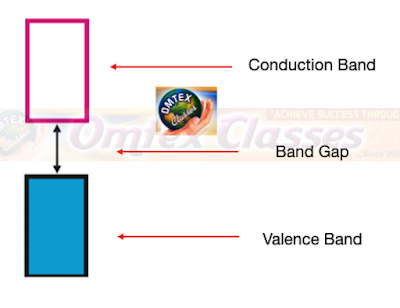# Answer the following in one or two sentences.

## A solid is hard, brittle and electrically nonconductor. Its melt conducts electricity. What type of solid is it?

Ionic solids are hard and brittle. They are nonconductors of electricity in solid-state. However, they are good conductors when melted. Hence, the given solid is an ionic solid.

# Answer the following in one or two sentences.

## Mention two properties that are common to both hcp and ccp lattices.

Properties common to both hcp and ccp lattice are the same coordination number of the particles (i.e., 12) and the same packing efficiency (74%).

# Answer the following in one or two sentences.

## Sketch a tetrahedral void.

Tetrahedral void: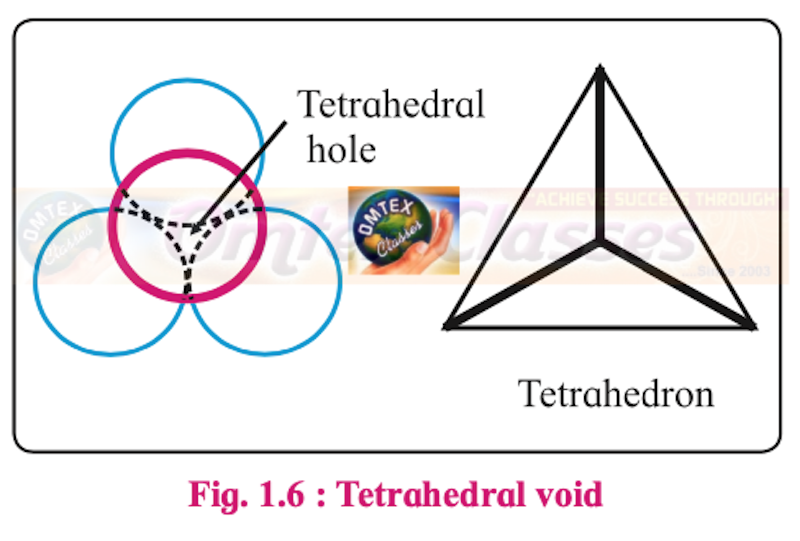# Answer the following in one or two sentences.

## What are ferromagnetic substances?

The substances containing large number of unpaired electrons are attracted strongly by magnetic field. Such substances are called as ferromagnetic substances.

# Answer the following in brief.

## What are valence band and conduction band?

#### Conduction band:

The highest energy band formed by the interaction of the outermost energy levels of closely spaced atoms in solids is called the conduction band.
The conduction band may be partially occupied or vacant.
Electrons in the conduction band are mobile and delocalized over the entire solid. They conduct electricity when an electrical potential is applied.

#### Valence band:

The band having lower energy than conduction band is the valence band.
The electrons in valence band are not free to move because they are tightly bound to the respective nuclei.

# Answer the following in brief.

## Distinguish between ionic solids and molecular solids

 Ionic solids Molecular solids 1. The constituent particles are charged ions (cations and anions). 1. The constituent particles are monoatomic or polyatomic molecules 2. The constituent particles are held by the electrostatic force of attraction between oppositely charged ions. 2. The constituent particles are held by intermolecular forces of attractions such as London forces, dipole-dipole forces and/or hydrogen bonds. 3. They are hard and brittle. 3. They are soft. 4. They have high melting points. 4. They have low melting points. 5. They are nonconductors of electricity in solid-state. However, they are good conductors when melted or dissolved in water. 5. They are poor conductors of electricity. 6. e.g. NaCl, CaF2, etc. 6. Ice, benzoic acid, etc.

# Answer the following in brief.

## Calculate the number of atoms in fcc unit cell.

A face-centred cubic (fcc) unit cell has particles at the eight corners plus particles at the centre of its six faces.

Each particle present at the corner of a given unit cell is shared with seven other neighbouring unit cells. As a result, its contribution to the given unit cell is only  ⅛.
Thus, the number of particles present at corners per unit cell
= 8 corner atoms × ⅛  atom per unit cell = 1

Each particle at the centre of the six faces is shared with one neighbouring cube. Thus, 1/2 of each face particle belongs to the given unit cell.
Thus, the number of particles present at faces per unit cell
= 6 atoms at the faces × ½ atom per unit cell = 3

Therefore, fcc unit cell has one corner particle plus 3 face particles, making total of 4 particles per unit cell.

# Answer the following in brief.

## How are the spheres arranged in first layer of simple cubic close-packed structures? How are the successive layers of spheres placed above this layer?

Simple cubic close-packed structures are obtained by stacking two-dimensional square close-packed layers.

So, the spheres in the first layer are placed adjacent to one another to form two dimensional square close packing (i.e., AAAA type arrangement). All the spheres of the successive layers are placed exactly above the spheres of the lower layers. This results in perfect horizontal and vertical alignment between the spheres of various layers.

# Answer the following in brief.

## Calculate the packing efficiency of metal crystal that has simple cubic structure.

Packing efficiency of metal crystal in the simple cubic lattice: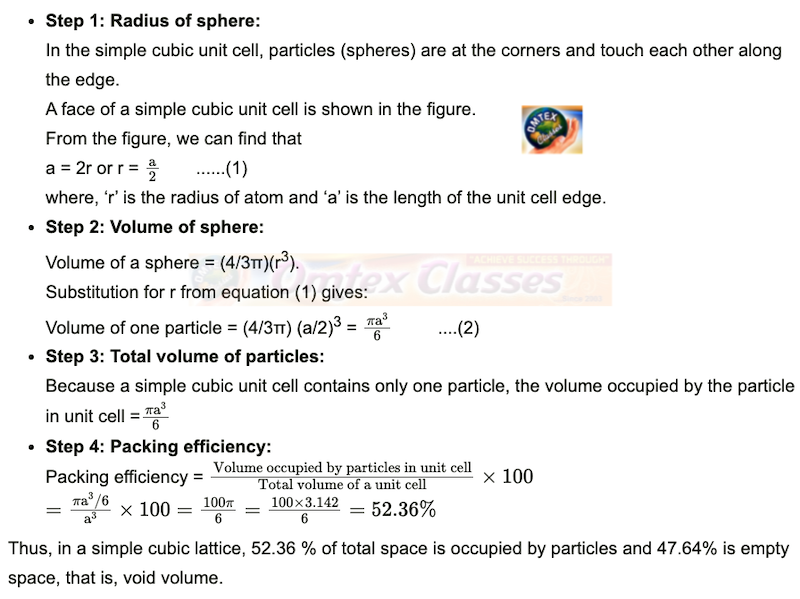# Answer the following in brief.

## What are paramagnetic substances? Give examples.

The substances with unpaired electrons are weakly attracted by the magnetic field. Such substances are called as paramagnetic substances.

e.g. Oxygen, Cu2+, Fe3+, Cr3+, etc

# Answer the following in brief.

## What are the consequences of Schottky defect?

Consequences of Schottky defect:

i. As the number of ions decreases, mass decreases. However, the volume remains unchanged. Hence, the density of a substance decreases.

ii. The number of missing cations and anions is equal. Hence, the electrical neutrality of the compound is preserved.

# Answer the following in brief.

## Cesium chloride crystallizes in a cubic unit cell with Cl– ions at the corners and a Cs+ ion in the centre of the cube. How many CsCl molecules are there in the unit cell?

Given: Cl ions are present at corners of the cube. Cs+ ion is at the centre of the cube.

To find: Number of CsCl molecules in the unit cell

Calculation:

i. Cl ions are present at the 8 corners. The contribution of each corner particle to the unit cell is 1/8. Hence, the number of Cl ions that belongs to the unit cell = 8 × (1/8) = 1

ii. Cs+ ion is at the centres of unit cell. The contribution of a particle at the centre to the unit cell is 1. Hence, the number of Cs+ ions that belongs to the unit cell = 1

There is one Cl ion and one Cs+ ion in the unit cell.

Hence, the number of CsCl molecules in the unit cell = 1.

Number of CsCl molecules in the unit cell = 1.

# Answer the following in brief.

## Cu crystallizes in fcc unit cell with edge length of 495 pm. What is the radius of Cu atom?

Given: Type of unit cell is fcc.

Edge length of unit cell (a) = 495 pm

To find: Radius of Cu atom (r)

Formula: For fcc unit cell, r = 0.3535 a

Calculation: Using formula,

r = 0.3535 a

= 0.3535 × 495 pm = 175 pm

Radius of Cu atom (r) is 175 pm.

# The density of iridium is 22.4 g/cm3. The unit cell of iridium is fcc. Calculate the radius of iridium atom. Molar mass of iridium is 192.2 g/mol.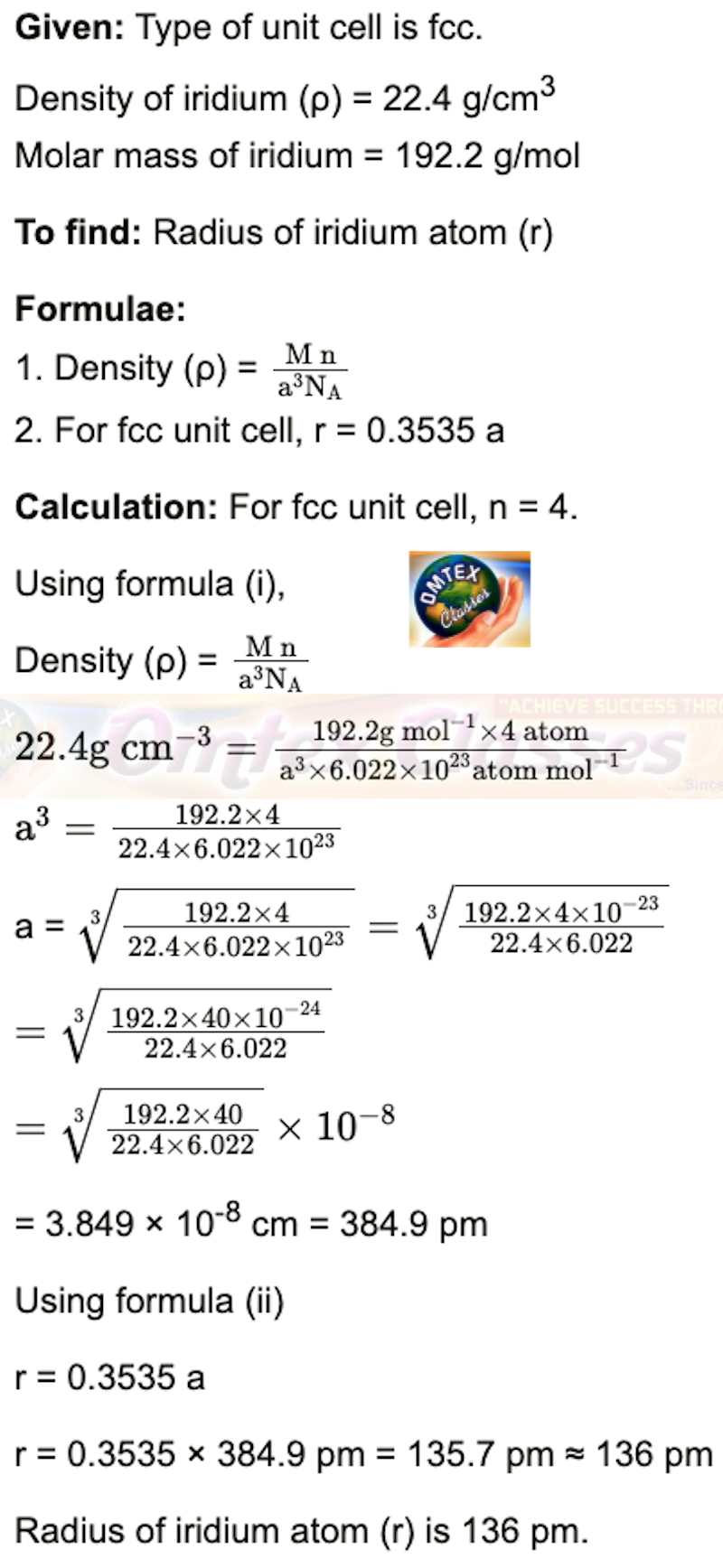# In ionic crystalline solid atoms of element Y form hcp lattice. The atoms of element X occupy one-third of tetrahedral voids. What is the formula of the compound?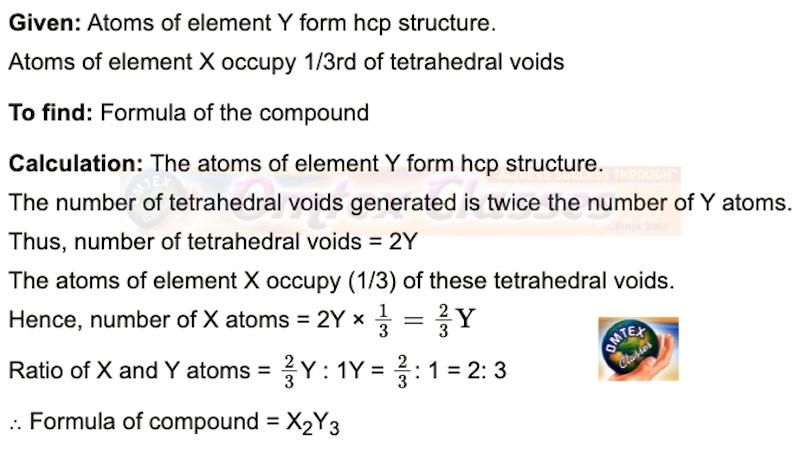# How are tetrahedral and octahedral voids formed?

Two dimensional hexagonal close-packed layer has triangular voids which is formed by three spheres.

When the triangular voids of the first layer are covered by spheres of the next layer, tetrahedral voids are formed. A tetrahedral void is surrounded by four spheres.

The overlapping triangular voids from the two layers together form an octahedral void.
An octahedral void is surrounded by six spheres.

Thus, the depressions in which spheres of second layer rest are tetrahedral voids while the depressions in which no sphere rests are octahedral voids.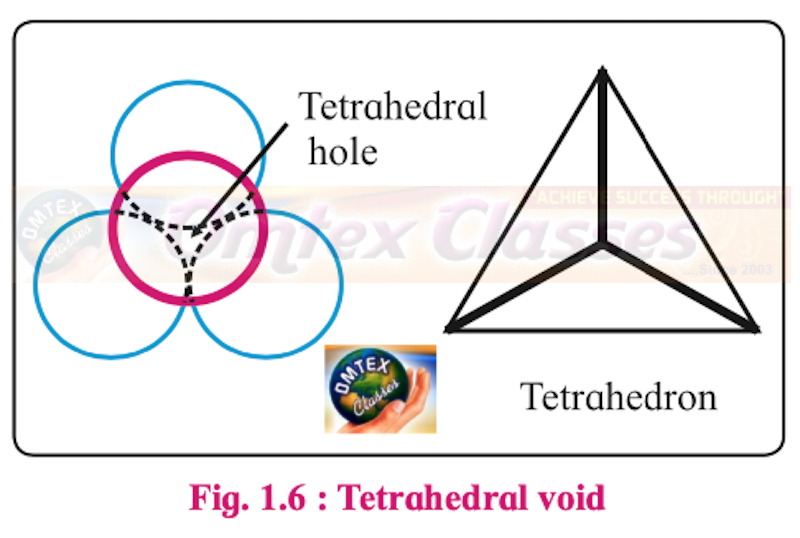# Third layer of spheres is added to second layer so as to form hcp or ccp structure. What is the difference between the addition of third layer to form these close-packed structures?

In ccp structure, the spheres of the third layer are not aligned with those of the first layer or second layer.

Hence, the third layer is called ‘C’ layer. The spheres of the fourth layer get aligned with the spheres of the first layer. Hence, the fourth layer is called ‘A’ layer. The resulting pattern of layers is called ‘ABCABC....’.

# An element with molar mass 27 g/mol forms a cubic unit cell with edge length of 405 pm. If the density of the element is 2.7 g/cm3, what is the nature of the cubic unit cell?# An element has a bcc structure with a unit cell edge length of 288 pm. How many unit cells and number of atoms are present in 200 g of the element? (Density of an element = 14.44 g/cm3 )# Distinguish with the help of diagrams metal conductors, insulators and semiconductors from each other.

 Metal conductors Insulators Semiconductors Metals are good conductors of electricity. Insulators are nonconductors of electricity. Semiconductors have conductivity that is intermediate between conductors and insulators. In metal conductors, the conduction band is partially filled and there is no band gap or there is overlapping between the valence band and conduction band. In insulators, the valence band and conduction band in insulators are separated by a large energy gap called the forbidden zone. In semiconductors, the valence band is completely filled with electrons, and the conduction band is empty. However, the energy gap between the two bands is smaller than that in an insulator.

# What are n-type semiconductors? Why is the conductivity of doped n-type semiconductor higher than that of pure semiconductor? Explain with diagram.

An extrinsic semiconductor, which is obtained by adding group 15 element to an intrinsic semiconductor which belongs to group 14, is called an n-type semiconductor.
e.g. Silicon doped with phosphorus

n-type semiconductor contains an increased number of electrons in the conduction band.

Consider the doping of Si with phosphorus. Si has a crystal structure in which each Si atom is linked tetrahedrally to four other Si atoms. When a small quantity of phosphorous is added to pure Si, the P atoms occupy some vacant sites in the lattice in place of Si atoms. The overall crystal structure of Si remains unchanged.
Four of the five valence electrons of P are utilized in bonding the closest to four Si atoms. Thus, P has one extra electron than needed for bonding. Therefore, Si doped with P has more number of electrons in the conduction band than those in the conduction band in pure Si. Thus, the conductivity of Si-doped with P is higher than that of pure Si. The electrons in the conduction band move under the influence of an applied potential and conduct electricity.

P atom occupying regular site of Si atom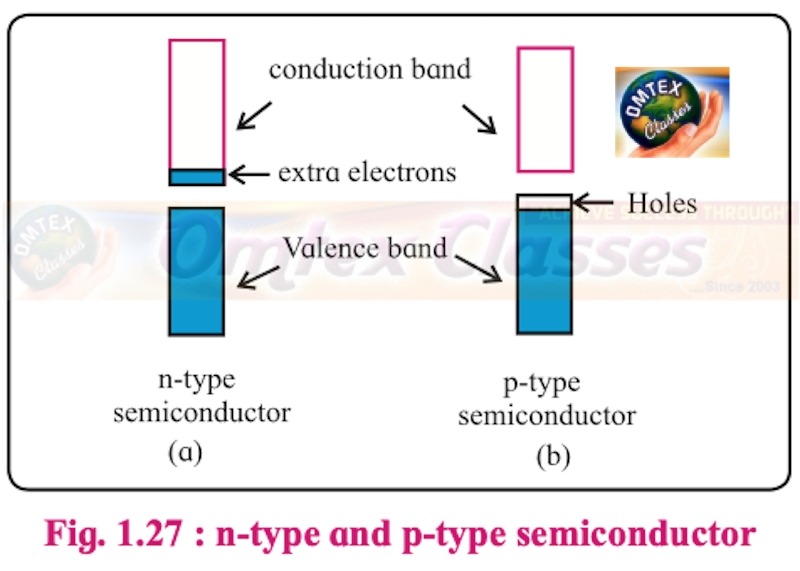# Explain with diagram, Frenkel defect. What are the conditions for its formation? What is its effect on density and electrical neutrality of the crystal?

#### Frenkel defect:

a. Frenkel defect arises when an ion of an ionic compound is missing from its regular lattice site and occupies an interstitial position between lattice points. The cations are usually smaller than anions. Therefore, the cations occupy interstitial sites.

b. The smaller cation is displaced from its normal site to interstitial space. It, therefore, creates a vacancy defect at its original position and an interstitial defect at its new location in the same crystal. Frenkel defect can be regarded as the combination of vacancy defect and interstitial defect.

c. This defect is found in ionic crystals like ZnS, AgCl, AgBr, AgI and CaF2.

#### Frenkel defect##### Conditions for the formation of Frenkel defect:

a. Frenkel defect occurs in ionic compounds with large difference between sizes of cation and anion.

b. The ions of ionic compounds must have low coordination number.

##### Consequences of Frenkel defect:

a. As no ions are missing from the crystal lattice as a whole, the density of solid and its chemical properties remain unchanged.

b. The crystal as a whole remains electrically neutral because the equal numbers of cations and anions are present.

# What is an impurity defect? What are its types? Explain the formation of vacancies through aliovalent impurity with example.

i. Impurity defect arises when foreign atoms, that is, atoms different from the host atoms, are present in the crystal lattice.

ii. There are two kinds of impurity defects: Substitutional and interstitial impurity defects.

iii. Formation of vacancy through aliovalent impurity:

Vacancies are created by the addition of impurities of aliovalent ions (that is, ions with oxidation state different from that of host ions) to an ionic solid.

e.g. Consider a small amount of SrCl2 impurity added to NaCl during its crystallization. The added Sr2+ ions (O.S. = +2) occupy some of the regular sites of Na+ host ions (O.S. = +1). In order to maintain electrical neutrality, every Sr2+ ion removes two Na+ ions. One of the vacant lattice sites created by the removal of two Na+ ions is occupied by one Sr2+ ion. The other site of Na+ ion remains vacant as shown in the figure.## PDF FILE TO YOUR EMAIL IMMEDIATELY PURCHASE NOTES & PAPER SOLUTION. @ Rs. 50/- each (GST extra)

SUBJECTS

HINDI ENTIRE PAPER SOLUTION

MARATHI PAPER SOLUTION
SSC MATHS I PAPER SOLUTION
SSC MATHS II PAPER SOLUTION
SSC SCIENCE I PAPER SOLUTION
SSC SCIENCE II PAPER SOLUTION
SSC ENGLISH PAPER SOLUTION
SSC & HSC ENGLISH WRITING SKILL
HSC ACCOUNTS NOTES
HSC OCM NOTES
HSC ECONOMICS NOTES
HSC SECRETARIAL PRACTICE NOTES

2019 Board Paper Solution

HSC ENGLISH SET A 2019 21st February, 2019

HSC ENGLISH SET B 2019 21st February, 2019

HSC ENGLISH SET C 2019 21st February, 2019

HSC ENGLISH SET D 2019 21st February, 2019

SECRETARIAL PRACTICE (S.P) 2019 25th February, 2019

HSC XII PHYSICS 2019 25th February, 2019

CHEMISTRY XII HSC SOLUTION 27th, February, 2019

OCM PAPER SOLUTION 2019 27th, February, 2019

HSC MATHS PAPER SOLUTION COMMERCE, 2nd March, 2019

HSC MATHS PAPER SOLUTION SCIENCE 2nd, March, 2019

SSC ENGLISH STD 10 5TH MARCH, 2019.

HSC XII ACCOUNTS 2019 6th March, 2019

HSC XII BIOLOGY 2019 6TH March, 2019

HSC XII ECONOMICS 9Th March 2019

SSC Maths I March 2019 Solution 10th Standard11th, March, 2019

SSC MATHS II MARCH 2019 SOLUTION 10TH STD.13th March, 2019

SSC SCIENCE I MARCH 2019 SOLUTION 10TH STD. 15th March, 2019.

SSC SCIENCE II MARCH 2019 SOLUTION 10TH STD. 18th March, 2019.

SSC SOCIAL SCIENCE I MARCH 2019 SOLUTION20th March, 2019

SSC SOCIAL SCIENCE II MARCH 2019 SOLUTION, 22nd March, 2019

XII CBSE - BOARD - MARCH - 2019 ENGLISH - QP + SOLUTIONS, 2nd March, 2019

HSC Maharashtra Board Papers 2020

(Std 12th English Medium)

HSC ECONOMICS MARCH 2020

HSC OCM MARCH 2020

HSC ACCOUNTS MARCH 2020

HSC S.P. MARCH 2020

HSC ENGLISH MARCH 2020

HSC HINDI MARCH 2020

HSC MARATHI MARCH 2020

HSC MATHS MARCH 2020

SSC Maharashtra Board Papers 2020

(Std 10th English Medium)

English MARCH 2020

HindI MARCH 2020

Hindi (Composite) MARCH 2020

Marathi MARCH 2020

Mathematics (Paper 1) MARCH 2020

Mathematics (Paper 2) MARCH 2020

Sanskrit MARCH 2020

Important-formula

THANKS